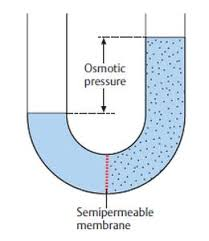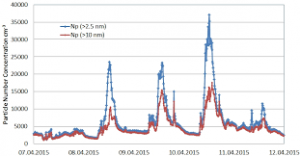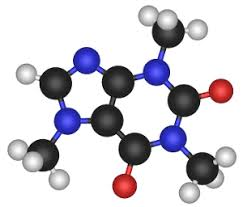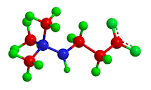## How to Calculate and Solve for Temperature, Number of Moles, Volume, Van’t Hoff Factor and Osmotic Pressure | The Calculator EncyclopediaThe image above represents the osmotic pressure.

To compute for the osmotic pressure, five parameters are needed and these parameters are Ideal Gas Constant (R)Temperature in Kelvin (T), Number of Moles (n), Volume (V) and Van’t Hoff’s Factor (i).

The formula for calculating osmotic pressure:

π = i nRTV

Where;
π = osmotic pressure
n = number of moles
R = ideal gas constant
T = temperature in Kelvin
i = Van’t Hoff’s Factor
V = Volume

Let’s solve an example;
Find the osmotic pressure when the ideal gas constant is 0.08206 with a temperature in kelvin of 120, number of moles is 32, a volume of 48 and a van’t hoff’s factor of 24.

This implies that;
n = number of moles = 32
R = ideal gas constant = 0.08206
T = temperature in Kelvin = 120
i = Van’t Hoff’s Factor = 24
V = Volume = 48

π = i nRTV
π = 24 32 x 0.08206 x 12048
π = (24) (315.110)(48)
π = (24)(6.5647)
π = 157.5

Therefore, the osmotic pressure is 157.5 atm.

Calculating the Van’t Hoff’s Factor using the Osmotic Pressure, Number of Moles, Temperature in Kelvin, Ideal Gas Constant and Volume.

i = / nRT

Where;
i = Van’t Hoff’s Factor
π = osmotic pressure
V = Volume
n = number of moles
R = ideal gas constant
T = temperature in Kelvin

Let’s solve an example;
Find the Van’t Hoff’s Factor when the osmotic pressure is 220, volume of 50, temperature in kelvin of 180 and number of moles of 60. (R = 0.08206)

This implies that;
π = osmotic pressure = 220
V = Volume = 50
n = number of moles = 60
R = ideal gas constant = 0.08206
T = temperature in Kelvin = 180

i = / nRT
i = 50 x 220 / 60 x 0.08206 x 180
i = 11000 / 866.808
i = 12.69

Therefore, the Van’t Hoff’s Factor is 12.69.

Calculating the Volume using the Osmotic Pressure, Number of Moles, Temperature in Kelvin, Ideal Gas Constant and Van’t Hoff’s Factor.

V = i (nRT) / π

Where;
V = Volume
i = Van’t Hoff’s Factor
π = osmotic pressure
n = number of moles
R = ideal gas constant
T = temperature in Kelvin

Let’s solve an example;
Find the volume when the osmotic pressure is 280, Van’t Hoff’s Factor of 40, temperature in kelvin of 90 and number of moles of 70. (R = 0.08206)

This implies that;
i = Van’t Hoff’s Factor = 40
π = osmotic pressure = 280
n = number of moles = 70
R = ideal gas constant = 0.08206
T = temperature in Kelvin = 90

V = i (nRT) / π
V = 40 (70 x 0.08206 x 90) / 280
V = 40 (516.978) / 280
V = 20679.12 / 280
V = 73.854

Therefore, the volume is 73.854.

## How to Calculate and Solve for the Number of Moles, Volume and Molar Concentration in Chemistry | Nickzom CalculatorThe image above represents the molar concentration.

To compute the molar concentration of a substance, two essential parameters are needed and these parameters are number of moles (n) and volume (V).

The formula for calculating the molar concentration:

c = nV

Where;
c = Molar Concentration
n = Number of moles
V = Volume

Let’s solve an example;
Find the molar concentration when the number of moles is 32 with a volume of 120.

This implies that;
n = Number of moles = 32
V = Volume = 120

c = nV
c = 32120
c = 0.266

Therefore, the molar concentration is 0.266 mol/L.

Calculating the Number of Moles when Molar Concentration and Volume is Given.

n = Vc

Where;
n = Number of moles
c = Molar Concentration
V = Volume

Let’s solve an example;
Find the number of moles when the molar concentration is 40 with a volume of 31.

This implies that;
c = molar concentration = 40
V = Volume = 31

n = Vc
n = 31 x 40
n = 1240

Therefore, the Number of Moles is 1240 mol.

## How to Calculate and solve for the Mass, Number of Moles and Molar Mass in Chemistry | The Calculator EncyclopediaThe image above represents the molar mass of a substance.

To compute for the molar mass, two essential parameters are needed and these parameters are mass (m) and number of moles (n).

The formula for calculating the molar mass:

M = mn

Where;
M = Molar mass
m = Mass
n = Number of moles

Let’s solve an example;
Find the molar mass of a substance with a mass of 14 kg and number of moles of 28 mol.

This implies that;
m = Mass = 14
n = Number of moles = 28

M = mn
M = 14 ⁄ 28
M = 0.5

Therefore, the molar mass is 0.5 Kg/mol.

Calculating the Mass of a Substance when the Molar Mass and the Number of Moles is Given.

m = nM

Where;
m = Mass
n = Number of moles
M = Molar Mass

Let’s solve an example;
Find the mass of a substance with a molar mass of 30 Kg/mol and number of moles of 15 mol.

This implies that;
n = Number of moles = 15
M = Molar Mass = 30

m = nM
m = 15 x 30
m =  450

Therefore, the mass of a substance is 450 kg.

## How to Calculate and Solve for the number of Particles in a Substance and Number of Moles | The Calculator EncyclopediaThe image above represent number of particles in a substance.

To compute for the number of particles in a substance, two essential paramters are needed and these parameters are Avogadro’s constant (h) and number of moles (n).

The formula for calculating the number of particles in a substance:

Number of particles in a substance = n x Avogadro’s constant

Where;
N  = Number of particles in a substance
n = Number of moles

Let’s solve an example:
Find the number of particles in a substance with an Avogadro’s constant of 6.022e+23 and number of moles of 12.

This implies that;
n = Number of moles = 12
h = Avogadro’s constant = 6.022e+23

Number of particles in the substance = 12 x 6.022e+23
Number of particles in the substance = 7.2264e+24

Therefore, the number of particles in the substance is 7.2264e+24 particles.

## Nickzom Calculator Solves Molar Concentration in Chemistry

According to Wikipedia,

Molar concentration (also called molarityamount concentration or substance concentration) is a measure of the concentration of a chemical species, in particular of a solute in a solution, in terms of amount of substance per unit volume of solution. In chemistry, the most commonly used unit for molarity is the number of moles per litre, having the unit symbol mol/L. A solution with a concentration of 1 mol/L is said to be 1 molar, commonly designated as 1 M.

Nickzom Calculator+ (Professional Version) – The Calculator Encyclopedia requires two parameters to get the answer for a molar concentration in Chemistry such as:

• Number of Moles
• Volume

One can access Nickzom Calculator+ (Professional Version) – The Calculator Encyclopedia via any of these channels:

First, go to the calculator map, scroll down to the Chemistry section and click on Basic Chemistry.Now, click on Molar Concentration in Chemistry